The Works of Jerry Iuliano  Ancient Numbers Revealed in Scientific Formulas   Compiled by Joseph E. Mason

Nollert's Constant and Loop Quantum Gravity
(Black Holes and the Beast 666)

In an article by J. Baez :

. . . are the mathematics for the most striking consequence of loop quantum gravity, that is the area of the singularity surface is the " soul " of the energy black hole ( 666 ) not the volume as in Newtonian gravity. According to Baez the Barrbero-Immirzi parameter ,

4 * ( IN 2 ) , is the key to the quantum areas of spin net-work edging. Baez states , after some mathematics that...." And now for a miracle ! A non-rotating black hole will exhibit damped oscillations when you perturb it momentarily in any way , and there are different vibrational modes called quasi-normal modes, each with its own characteristic frequency and damping. In 1993 Nollert used computer calculations to show that in the limit of large damping, the frequency of these modes approaches a specific number depending only on the mass of the black hole . . . :
 W = .04371235 / M

. . . plugging this into the previous formula, Hod in 1994 obtained the quantum of the area:

 quantum A = 4.394444 ~ 4 * (IN 3 )

. . . end Baez quote." . . . Later it was shown that the quantum area is indeed , 4* ( IN 3 ).This form is ancient in text as can be demonstrated through the collective unconscious form:

 IN 81 = IN 9 + IN 9 = 4 * ( IN 3 )

 IN( 80 * ( 666 ^ ( .04371235 ^ 2 ))) = 4 * ( IN 3 )

. . . the answer to what the composition of 80 is, can be shown as a complex variable relating to the following constants: unification of product and sum

 80 + 1 = 80 * ( 666 ^ ( .0437124445 ^ 2 ))

 h = Planck's constant = 6.6260693 * ( 10 ^ - 34 ) J  c= speed of light = 299792458 m/s  Gn = gravitational constant = 6.67420559 * ( 10 ^ - 11 ) m^3/kg/s^2  Mp = Planck mass = 2.176449596 * ( 10 ^ - 8 ) kg  Me = electron mass = 9.109383749 * ( 10 ^ -31 ) kg  Mpr = proton mass = 1.672621709 * ( 10 ^ - 2 7) kg  Gw = fermi coupling constant , weak force = .00001166403038 F/m    Gf = neutrino bosonic = 3.685861966 * ( 10 ^ - 82 ) F/m  Fd = Feigenbaum delta constant = 4.669201609

. . . the electron - proton mass ratio:

 (( h * c / Pi ) ^ 3 ) * Me / Mpr / Gf / F = 80

. . . the weak force fermi-coupler is the ratio of the electron-proton masses:

 Me / Mpr = Gw * F

. . . which then rewrites to :

 (( h * c / Pi ) ^ 3 ) * Gw / Gf * 10 = 80

. . . but Gw/Gf can rewrite to:

 1 / ( Gn ^ 3 ) / ( Mp ^ 6 ) = Gw / Gf

...which is by substitution for gravity , Gn :

 (( h * c / Gn ) ^ 3 ) * 10 / ( Mp ^ 6 ) = 80

. . . since ( Gn^3) * ( Mp^6 ) = Gf / Gw then the gravity form can be rewritten:

 (( h * c / Pi ) ^ 3 ) * 10 / ( Gn ^ 3 ) / ( Mp ^ 6 ) = 80

 (( h * c / Pi ) ^ 3 ) * 10 * Gw / Gf = 80

. . . the Beast is the black hole both symbolically and literally (mathematical) As mathematical proof can be shown by the amazing equality that simplifies everything to its minimum. That is, if one states that the black hole form is literally(mathematically)

represented by the symbolic number 666 and the frequency to mass constant that always occurs in black hole singularities: Nollert's constant . . . 04371235

 Wfrequency = .04371235 / Mmass  Wfrequency * Mmass = .04371235

. . . is attached exponentially ( ^ ) to the black hole singularity as energy, or frequency squared , then the result is a perfect rational integer, which suggests quantum gravitational frequencies of perfect integer modules ....mod 1/80

 666 ^ (( Wfrequency*Mmass) ^ 2 ) = 1 + ( 1/ 80 )                    .0437124445

The collective unconscious exists as a function of this black hole (666) through the electro-magnetic (carnal) rules , guided by the amplitude for matter(electron) to change into energy(photon) . . . aem = fine-structure constant = 1/137.03599911 . . . note the module connection of 1/80 to 144 through one-half the variables of the Beast . . .

 (666)37 * 18 / ( 10 ^ ( 143.9999879 / 37 )) = sqrt aem = sqrt 137.0359994   144 * 1/ 80 = 1.8

. . . or one-half the Beast factor . . . 37 * 144 * 1/80 = 66.6

-----

From the J.Baez article . . .

" And now for the miracle! A nonrotating black hole will exhibit damped oscillations when you perturb it momentarily in any way, and there are different vibrational modes called quasinormal modes, each with its own characteristic frequency and damping. In 1993, Nollert  used computer calculations to show that in the limit of large damping, the frequency of these modes approaches a specific number depending only on the mass of the black hole: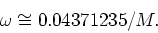Plugging this into the previous formula, Hod obtained the quantum of area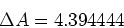and noticed that this was extremely close to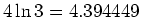On the basis of this, he daringly concluded that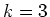Our story now catches up with recent developments. In November 2002, Dreyer  found an ingenious way to reconcile Hod's result with the loop quantum gravity calculation. The calculation due to Ashtekar et al used a version of loop quantum gravity where the gauge group is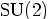This is why so many formulas resemble those familiar from the quantum mechanics of angular momentum, and this is why the smallest nonzero area comes from a spin network edge labeled by the smallest nonzero spin: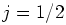But there is also a version of loop quantum gravity with gauge group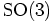in which the smallest nonzero spin is . Dreyer observed out that if we repeat the black hole entropy calculation using thistheory, we get a quantum of area that matches Hod's result! One can easily check this by redoing the calculation sketched earlier, replacingby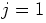. One finds a new value of the Immirzi parameter: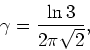and obtains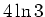as the new quantum of area. But ultimately, all that really matters is that whenthere are 3 spin states instead of 2. Thus each quantum of area carries a `trit' of information instead of a bit, which is why Dreyer obtainsWith the appearance of Dreyer's paper, the suspense became almost unbearable. After all, Hod's observation relied on numerical calculations, so the very next digit of his number might fail to match that of.

Luckily, in December 2002, Motl  showed that the match is exact! He used an ingenious analysis of Nollert's continued fraction expansion for the asymptotic frequencies of quasinormal modes.

While exciting, these developments raise even more questions than they answer. Why shouldloop quantum gravity be the right theory to use? After all, it seems impossible to couple spin-1/2 particles to this version of the theory. Corichi has sketched a way out of this problem , but much work remains to see whether his proposal is feasible. Can we turn Hod's argument from a heuristic into something a bit more rigorous? He cites Bohr's correspondence principle in this form: ``transition frequencies at large quantum numbers should equal classical oscillation frequencies.'' However, this differs significantly from the idea behind Bohr-Sommerfeld quantization, and it is also unclear why we should apply it only to the asymptotic frequencies of highly damped quasinormal modes. Can the mysterious agreement betweenloop quantum gravity and Hod's calculation be extended to rotating black holes? Here a new paper by Hod makes some interesting progress . Can it be extended to black holes in higher dimensions? Here Motl's new work with Neitzke gives some enigmatic clues . Stay tuned for further developments.

J. Iuliano
(Sent via e-mail August 1, 2006)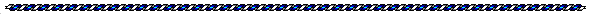Help PagesLinks to other articles in the series -

The Works of Jerry Iuliano
Ancient Numbers Revealed in Scientific FormulasTHETA – UNITY EQUATIONS
by Jerry Iuliano

Ancient Mysteries Article
by Jerry Iuliano

Electronic Gravity
by Jerry Iuliano

Why Study Metrology?
by Livio C. Stecchini
". . . all important units of weight and volume exist in
two varieties related as 24:25 or 96:100."

82944 & the Four Fundamental Forces & the God Particle
by Dr. D. G. Leahy

Earth/matriX
Charles Johnson

A Mathematical Constant: 288
by Charles Johnson

A History of Measures
by Livio C. Stecchini

Richard Feynman: The Douglas Robb Memorial Lectures
streaming video from 1979